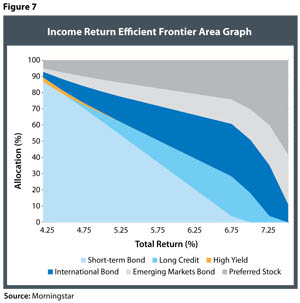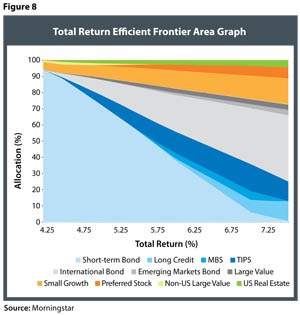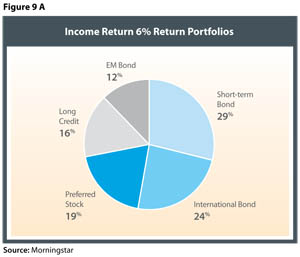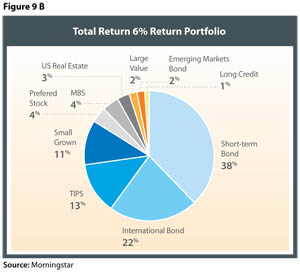# Efficient Income Investing

December 21, 2012

Optimisation Results

In this section we explore the differences that result from optimising a portfolio using the traditional total return approach (based on equation 1) versus one in which we use an income approach (equation 2).

Figures 7 and 8 demonstrate the different portfolio allocations in an “area graph” format, based on income return and total return, respectively, for total returns ranging from 4.25 percent to 7.50 percent. The income return efficient frontiers were constructed using an approach that focuses on income return rather than total return, but the portfolios are contrasted from a total return perspective so that the reader can better understand the respective differences in the approaches, given the same overall target return.Both optimisation approaches feature heavy allocations to short-term bonds for the lower return portfolios. However, there are considerable differences in the non-short-term portion. There are a number of asset classes that are featured prominently in the income return portfolios that do not appear in the total return portfolios (and vice versa). For example, the only equity component in the income return portfolios in Figure 7 is preferred stock, while the total return portfolios feature a number of different asset classes, such as large value, small growth, and US real estate. To give the reader a more precise example of the differences in the two approaches, Figure 9 has been included, which compares the optimal allocations for the income return and total return methodologies, given a total return of 6 percent.

The total return portfolios are more diversified than the income return portfolios. The likely reason for this is that the total return portfolios are focused on total return and are indifferent to the source of that return. In the income portfolios, we seek to maximise income while minimising total risk. This additional restriction limits the feasible set. The information in Figures 7 and 9 suggests that an investor seeking to build an income-efficient portfolio should consider including asset classes such as preferred stock, emerging market bonds, international bonds, long-term bonds, and (potentially) short-term bonds to create efficient portfolios. An investor looking to maximise income within a diversified portfolio framework could substitute short-term bonds with high-yield bonds (for example) to increase the yield.